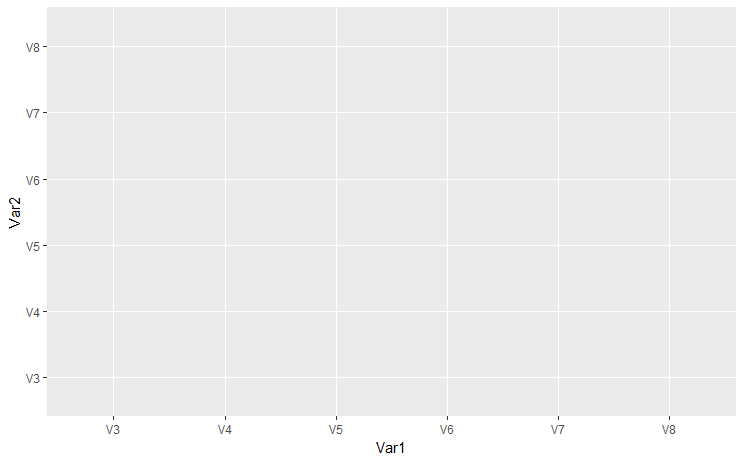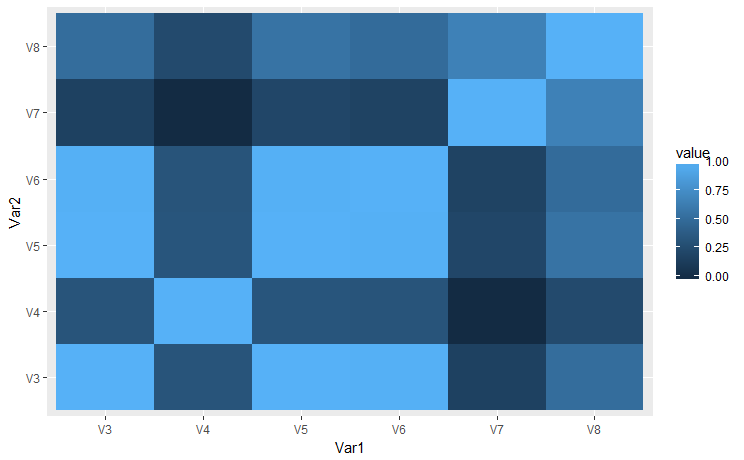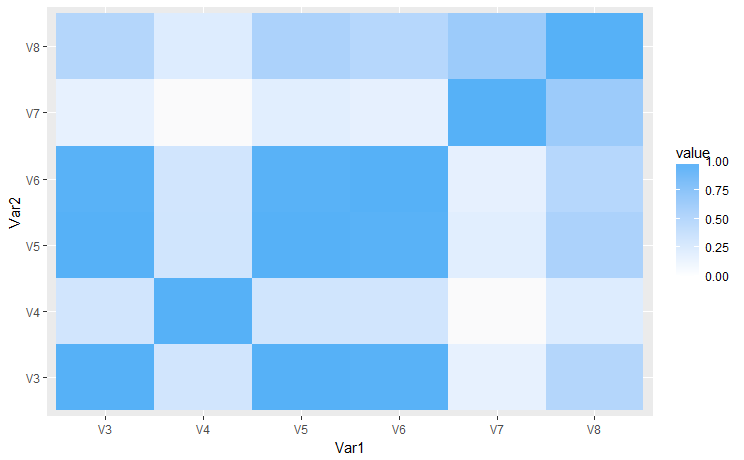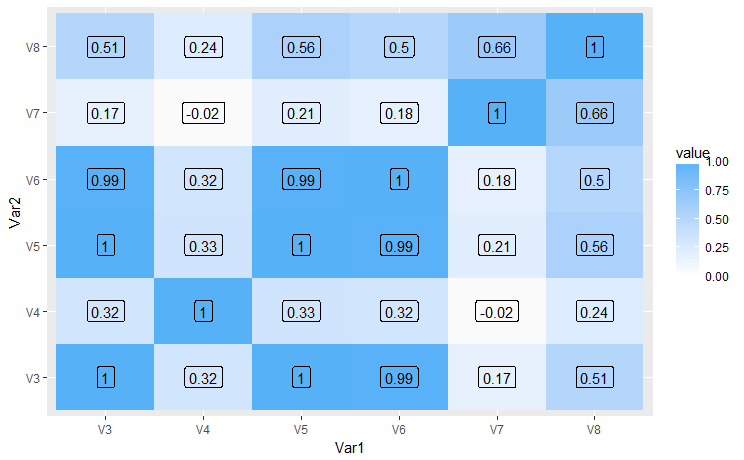# Correlation Matrix Heatmap in R

Hello friends! Wishing you all a Very Happy New Year 2018!

Today we’ll be seeing the correlation matrix heatmap. Heatmaps are visually appealing with quick and easy to get inference. Follow the quick and easy tutorial.

##### Install necessary R packages
```#----Install Packages
install.packages("ggplot2")
install.packages("reshape2")

library(reshape2)
library(ggplot2)```
```#Reading the data

#Read Column 3 to 8 and using it for correlation
data<- mydata[,3:8]```
##### Create Correlation Matrix

Once the correlation matrix is prepared it has to bring in proper format to plot in a chart.

```#Create correlation matrix
cordata <- round(cor(data),2)
head(cordata) #This will be a 5X5 matrix with each correlation values

#Melt data to bring the correlation values in two axis
melted_cordata <- melt(cordata)

##### Creating the Correlation matrix Heatmap step by step

Creating a basic background as shown below.

```plot <- ggplot(data = melted_cordata, aes(x=Var1, y=Var2, fill=value,
label= value))```Adding layers to the base

`plot_tile <- plot + geom_tile()`Adding the color scale to other than default

```plot_fill_color <- plot_tile + scale_fill_gradient2(low = "#132B43",high ="#56B1F7"
,mid = "white")```Adding correlation values(as label) to the plot

`plot_label <- plot_fill_color + geom_text()`If you want to add box to the label as shown in the image below.

`plot_label_box <- plot_label + geom_label()`There are more changes which can be done here like making it dynamic to choose the columns, adding tool tip, dynamic color scale etc. This was a basic intro about the correlation matrix heatmaps in R.

Sources and Read more

Keep visiting Analytics Tuts for more tutorials.

Thanks for reading! Comment your suggestion and queries.